# Stockmayer potential

Jump to: navigation, search

The Stockmayer potential consists of the Lennard-Jones model with an embedded point dipole. Thus the Stockmayer potential becomes (Eq. 1 ):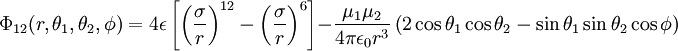$\Phi_{12}(r, \theta_1, \theta_2, \phi) = 4 \epsilon \left[ \left(\frac{\sigma}{r} \right)^{12}- \left( \frac{\sigma}{r}\right)^6 \right] - \frac{\mu_1 \mu_2}{4\pi \epsilon_0 r^3} \left(2 \cos \theta_1 \cos \theta_2 - \sin \theta_1 \sin \theta_2 \cos \phi\right)$

where:

•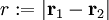$r := |\mathbf{r}_1 - \mathbf{r}_2|$
•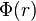$\Phi(r)$ is the intermolecular pair potential between two particles at a distance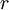$r$
•$\sigma$ is the diameter (length), i.e. the value of$r$ at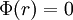$\Phi(r)=0$
•$\epsilon$ represents the well depth (energy)
•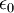$\epsilon_0$ is the permittivity of the vacuum
•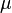$\mu$ is the dipole moment
•$\theta_1$ and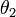$\theta_2$ are the angles associated with the inclination of the two dipole axes with respect to the intermolecular axis.
•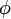$\phi$ is the azimuth angle between the two dipole moments

If one defines a reduced dipole moment,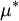$\mu^*$, such that: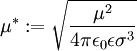$\mu^* := \sqrt{\frac{\mu^2}{4\pi\epsilon_0\epsilon \sigma^3}}$

one can rewrite the expression as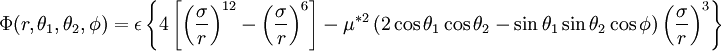$\Phi(r, \theta_1, \theta_2, \phi) = \epsilon \left\{4\left[ \left(\frac{\sigma}{r} \right)^{12}- \left( \frac{\sigma}{r}\right)^6 \right] - \mu^{*2} \left(2 \cos \theta_1 \cos \theta_2 - \sin \theta_1 \sin \theta_2 \cos \phi\right) \left(\frac{\sigma}{r} \right)^{3} \right\}$

For this reason the potential is sometimes known as the Stockmayer 12-6-3 potential.

## Critical properties

In the range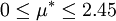$0 \leq \mu^* \leq 2.45$ :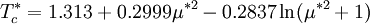$T_c^* = 1.313 + 0.2999\mu^{*2} -0.2837 \ln(\mu^{*2} +1)$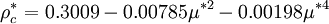$\rho_c^* = 0.3009 - 0.00785\mu^{*2} - 0.00198\mu^{*4}$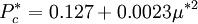$P_c^* = 0.127 + 0.0023\mu^{*2}$

## Bridge function

A bridge function for use in integral equations has been calculated by Puibasset and Belloni .